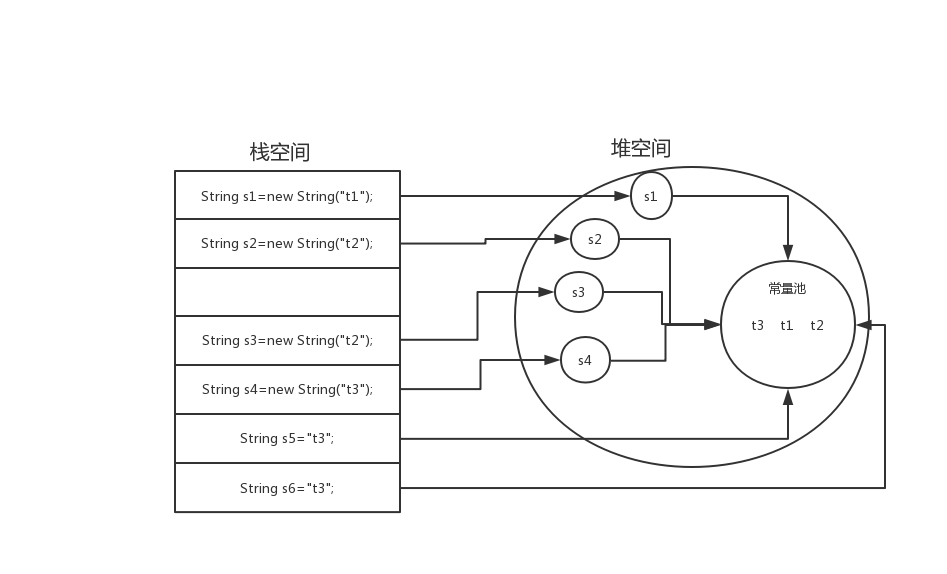# Java的==和equals

## 首先看看Java的栈空间和堆空间的地址引用## ==的说明

public class demo2 {
public static void main(String[] args) {
String s1=new String("t1");
String s2=new String("t2");
String s3=new String("t2");

String s4=new String("t3");
String s5="t3";
String s6="t3";

System.out.println("s2==s3:"+(s2==s3));
System.out.println("s5==s6:"+(s5==s6));
}
}


## equals的说明

public boolean equals(Object anObject) {
if (this == anObject) {
return true;
}
if (anObject instanceof String) {
String anotherString = (String)anObject;
int n = value.length;
if (n == anotherString.value.length) {
char v1[] = value;
char v2[] = anotherString.value;
int i = 0;
while (n-- != 0) {
if (v1[i] != v2[i])
return false;
i++;
}
return true;
}
}
return false;
}

• 首先它会对比地址，如果地址相等，就直接返回true
• 如果地址不相等，就会对比里面的每一个字符，直到完全相等，然后返回true

## 总结

所以一般如果是对比两个对象是否相等的话，用==就可以。但是如果你要对比两个值是否相等的话，就要用equals了，因为如果用==就会出现上面明明值相等,返回却是false的情况。